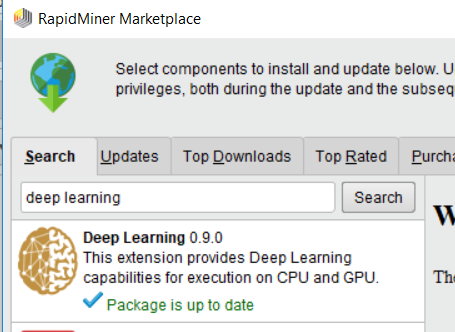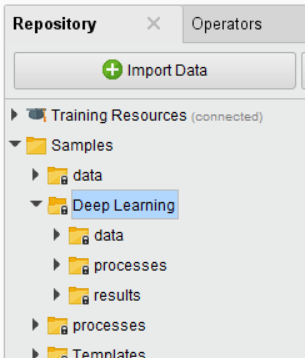# HOW TO APPLY DBScan & Deep Learning on Classification Model

Member Posts: 1Newbie
Hi,

I'm pretty new with RapidMiner and wondering how to apply DBScan & Deep Learning on a classification model.
My data is binomial. Do I need to use binomial or integer values for these methods?

• Moderator, Member Posts: 1,207Unicorn
Hi @kimchi

Deep learning in RM can be done using a deep learning extension that is available in the market place (Menu Bar --> Extensions --> Market Place). You need to install that.

If you are just trying to apply deep learning with fully connected layers you can use the deep learning operator that comes with RM, but if you are looking to apply CNN, LSTM then you need to have deep learning extension.

If you install a deep learning extension from market place it comes with examples. You can see below screenshots for understanding. I also added code for a deep learning operator which is a multilayer feed forward neural network.```<?xml version="1.0" encoding="UTF-8"?><process version="9.1.000">
<context>
<input/>
<output/>
<macros/>
</context>
<operator activated="true" class="process" compatibility="9.1.000" expanded="true" name="Process">
<parameter key="logverbosity" value="init"/>
<parameter key="random_seed" value="2001"/>
<parameter key="send_mail" value="never"/>
<parameter key="process_duration_for_mail" value="30"/>
<parameter key="encoding" value="SYSTEM"/>
<process expanded="true">
<operator activated="true" class="retrieve" compatibility="9.1.000" expanded="true" height="68" name="Retrieve Iris" width="90" x="45" y="238">
<parameter key="repository_entry" value="//Samples/data/Iris"/>
</operator>
<operator activated="true" class="concurrency:cross_validation" compatibility="9.1.000" expanded="true" height="166" name="Cross Validation" width="90" x="246" y="85">
<parameter key="split_on_batch_attribute" value="false"/>
<parameter key="leave_one_out" value="false"/>
<parameter key="number_of_folds" value="5"/>
<parameter key="sampling_type" value="automatic"/>
<parameter key="use_local_random_seed" value="false"/>
<parameter key="local_random_seed" value="1992"/>
<parameter key="enable_parallel_execution" value="true"/>
<process expanded="true">
<operator activated="true" class="h2o:deep_learning" compatibility="9.0.000" expanded="true" height="82" name="Deep Learning" width="90" x="179" y="34">
<parameter key="activation" value="Rectifier"/>
<enumeration key="hidden_layer_sizes">
<parameter key="hidden_layer_sizes" value="50"/>
<parameter key="hidden_layer_sizes" value="50"/>
</enumeration>
<enumeration key="hidden_dropout_ratios"/>
<parameter key="use_local_random_seed" value="false"/>
<parameter key="local_random_seed" value="1992"/>
<parameter key="epochs" value="10.0"/>
<parameter key="compute_variable_importances" value="false"/>
<parameter key="train_samples_per_iteration" value="-2"/>
<parameter key="epsilon" value="1.0E-8"/>
<parameter key="rho" value="0.99"/>
<parameter key="learning_rate" value="0.005"/>
<parameter key="learning_rate_annealing" value="1.0E-6"/>
<parameter key="learning_rate_decay" value="1.0"/>
<parameter key="momentum_start" value="0.0"/>
<parameter key="momentum_ramp" value="1000000.0"/>
<parameter key="momentum_stable" value="0.0"/>
<parameter key="standardize" value="true"/>
<parameter key="L1" value="1.0E-5"/>
<parameter key="L2" value="0.0"/>
<parameter key="max_w2" value="10.0"/>
<parameter key="loss_function" value="Automatic"/>
<parameter key="distribution_function" value="AUTO"/>
<parameter key="early_stopping" value="false"/>
<parameter key="stopping_rounds" value="1"/>
<parameter key="stopping_metric" value="AUTO"/>
<parameter key="stopping_tolerance" value="0.001"/>
<parameter key="missing_values_handling" value="MeanImputation"/>
<parameter key="max_runtime_seconds" value="0"/>
<list key="expert_parameters"/>
<list key="expert_parameters_"/>
</operator>
<connect from_port="training set" to_op="Deep Learning" to_port="training set"/>
<connect from_op="Deep Learning" from_port="model" to_port="model"/>
<portSpacing port="source_training set" spacing="0"/>
<portSpacing port="sink_model" spacing="0"/>
<portSpacing port="sink_through 1" spacing="0"/>
</process>
<process expanded="true">
<operator activated="true" class="apply_model" compatibility="9.1.000" expanded="true" height="82" name="Apply Model" width="90" x="45" y="34">
<list key="application_parameters"/>
<parameter key="create_view" value="false"/>
</operator>
<operator activated="true" class="multiply" compatibility="9.1.000" expanded="true" height="103" name="Multiply" width="90" x="112" y="187"/>
<operator activated="true" class="performance" compatibility="9.1.000" expanded="true" height="82" name="Performance" width="90" x="179" y="34">
<parameter key="use_example_weights" value="true"/>
</operator>
<operator activated="true" class="performance_classification" compatibility="9.1.000" expanded="true" height="82" name="Performance (2)" width="90" x="246" y="187">
<parameter key="main_criterion" value="first"/>
<parameter key="accuracy" value="true"/>
<parameter key="classification_error" value="false"/>
<parameter key="kappa" value="true"/>
<parameter key="weighted_mean_recall" value="false"/>
<parameter key="weighted_mean_precision" value="false"/>
<parameter key="spearman_rho" value="false"/>
<parameter key="kendall_tau" value="false"/>
<parameter key="absolute_error" value="false"/>
<parameter key="relative_error" value="false"/>
<parameter key="relative_error_lenient" value="false"/>
<parameter key="relative_error_strict" value="false"/>
<parameter key="normalized_absolute_error" value="false"/>
<parameter key="root_mean_squared_error" value="false"/>
<parameter key="root_relative_squared_error" value="false"/>
<parameter key="squared_error" value="false"/>
<parameter key="correlation" value="false"/>
<parameter key="squared_correlation" value="false"/>
<parameter key="cross-entropy" value="false"/>
<parameter key="margin" value="false"/>
<parameter key="soft_margin_loss" value="false"/>
<parameter key="logistic_loss" value="false"/>
<parameter key="skip_undefined_labels" value="true"/>
<parameter key="use_example_weights" value="true"/>
<list key="class_weights"/>
</operator>
<connect from_port="model" to_op="Apply Model" to_port="model"/>
<connect from_port="test set" to_op="Apply Model" to_port="unlabelled data"/>
<connect from_op="Apply Model" from_port="labelled data" to_op="Multiply" to_port="input"/>
<connect from_op="Multiply" from_port="output 1" to_op="Performance (2)" to_port="labelled data"/>
<connect from_op="Multiply" from_port="output 2" to_op="Performance" to_port="labelled data"/>
<connect from_op="Performance" from_port="performance" to_port="performance 1"/>
<connect from_op="Performance" from_port="example set" to_port="test set results"/>
<connect from_op="Performance (2)" from_port="performance" to_port="performance 2"/>
<portSpacing port="source_model" spacing="0"/>
<portSpacing port="source_test set" spacing="0"/>
<portSpacing port="source_through 1" spacing="0"/>
<portSpacing port="sink_test set results" spacing="0"/>
<portSpacing port="sink_performance 1" spacing="0"/>
<portSpacing port="sink_performance 2" spacing="0"/>
<portSpacing port="sink_performance 3" spacing="0"/>
</process>
</operator>
<connect from_op="Retrieve Iris" from_port="output" to_op="Cross Validation" to_port="example set"/>
<connect from_op="Cross Validation" from_port="example set" to_port="result 3"/>
<connect from_op="Cross Validation" from_port="test result set" to_port="result 2"/>
<connect from_op="Cross Validation" from_port="performance 1" to_port="result 1"/>
<connect from_op="Cross Validation" from_port="performance 2" to_port="result 4"/>
<portSpacing port="source_input 1" spacing="0"/>
<portSpacing port="sink_result 1" spacing="0"/>
<portSpacing port="sink_result 2" spacing="0"/>
<portSpacing port="sink_result 3" spacing="0"/>
<portSpacing port="sink_result 4" spacing="0"/>
<portSpacing port="sink_result 5" spacing="0"/>
</process>
</operator>
</process>
```

Thanks,
Varun

Regards,
Varun
https://www.varunmandalapu.com/